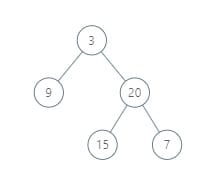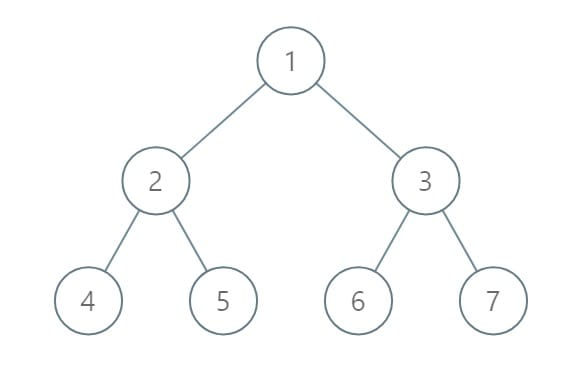# 987. Vertical Order Traversal of a Binary Tree

Reference: LeetCode
Difficulty: Medium

## Problem

Given a binary tree, return the vertical order traversal of its nodes values.
For each node at position (X, Y), its left and right children respectively will be at positions (X - 1, Y - 1) and (X + 1, Y - 1).
Running a vertical line from X = -infinity to X = +infinity, whenever the vertical line touches some nodes, we report the values of the nodes in order from top to bottom (decreasing Y coordinates).
If two nodes have the same position, then the value of the node that is reported first is the value that is smaller.
Return an list of non-empty reports in order of X coordinates. Every report will have a list of values of nodes.

Example:## Analysis

Methods:

1. HashMap + TreeMap + PQ (reference)
• Use a HashMap to record X, and record minX and maxX for future iteration.
• Why? Because HashMap gets the key in constant time, while TreeMap needs $O(\log{N})$.
• And also notice that the values of X is continuous. So we can iterate over the map easily if we have minX and maxX.
• Use a TreeMap to record Y, and use a PriorityQueue to keep nodes with same (x, y) in ascending order.
• Y is not continuous. We use keySet() in TreeMap to return keys in ascending order, so we can traverse all Y given a specific X.
• Since some nodes have the same position and we care about their ordering, we use PriorityQueue.
• It turns out that we can also use ArrayList, and the time complexity does not differ very much. If using ArrayList, remember to sort it manually.

Time: $O(N\log{N})$ for each node the runtime could be upper bounded by $O(\log{N})$.
Space: $O(N)$

Review code:

CommentJunhao Wang
a software engineering cat